Next: Special Symbols Up: Commands for Math Mode Previous: Subscripting and Superscripting

## Text in Math Mode

In math mode,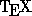ignores all spaces. It has complicated built-in rules which it uses to determine how the equation should be spaced. (For instance, it knows to put a little extra space around + and = signs.) But sometimes you want to put words in the middle of an equation. For instance, you might want to generate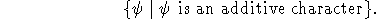The way you do this is to use the \text{ } command to tell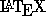to go back into text mode briefly. In our example, you would type

$$\{\psi \mid \psi \text{ is an additive character}\}$$.
Thenwill set is an additive character'' with a space before it in text mode. Note that if we had left out the space before is'', we would have gotThis brings up a point about using math mode properly. In math mode,assumes that any string of letters next to one another is a product, and spaces it accordingly. If, in fact, it was a word, it won't look good. So, for symbols which are actually parts of words, like Stab, Spec, Proj, etc., you should use \operatorname{ } to tellto space the letters in the symbol as text, not as a product. For instance, type

$$X := \operatorname{Spec}A$$
to get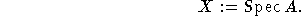You can make subscripts and superscripts of symbols you have generated using the \operatorname{ } command: e.g., $\operatorname{Stab}_G(H)$ gives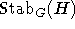.

Processed by LaTeX2html